# NCERT Solution Inequalities Class 11 Maths Chapter 6 Exercise 6.1

In this page we have NCERT Solution Inequalities Class 11 Maths Chapter 6 Exercise 6.1 . Hope you like them and do not forget to like , social share and comment at the end of the page.
Question 1:
Solve 24x < 100, when (i) x is a natural number (ii) x is an integer
Question 2:
Solve –12x > 30, when
(i) x is a natural number (ii) x is an integer
Question 3:
Solve 5x– 3 < 7, when
(i) x is an integer (ii) x is a real number
Question 4:
Solve 3x + 8 > 2, when
(i) x is an integer (ii) x is a real number
Question 5:
Solve the given inequality for real x: 4x + 3 < 5x + 7
Question 6:
Solve the given inequality for real x: 3(x – 1) ≤ 2 (x – 3)
Question 7:
Solve the given inequality for real x: 3x – 7 > 5x – 1
Question 8
Solve the given inequality for real x: 3(2 – x) ≥ 2(1 – x)
Question 9
Solve the given inequality for real x: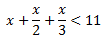Question 10
Solve the given inequality for real x: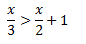Question 11
Solve the given inequality for real x: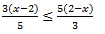Question 12:
Solve the given inequality for real x: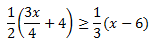Question 13:
Solve the given inequality for real x: 2(2x + 3) – 10 < 6 (x – 2)
Question 14
Solve the given inequality for real x: 37 – (3x + 5) ≥ 9x – 8(x – 3)
Question 15
Solve the given inequality for real x: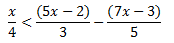Question 16
Solve the given inequality for real x: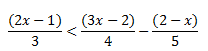Question 17
Solve the given inequality and show the graph of the solution on number line: 3x – 2 < 2x +1
Question 18
Solve the given inequality and show the graph of the solution on number line: 5x – 3 ≥ 3x – 5
Question 19
Solve the given inequality and show the graph of the solution on number line: 3(1 – x) < 2 (x + 4)
Question 20
Solve the given inequality and show the graph of the solution on number line: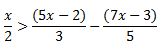Question 21:
Ravi obtained 70 and 75 marks in first two unit test. Find the minimum marks he should get in the third test to have an average of at least 60 marks.
Question 22:
To receive Grade ‘A’ in a course, one must obtain an average of 90 marks or more in five examinations (each of 100 marks). If Sunita’s marks in first four examinations are 87, 92, 94 and 95, find minimum marks that Sunita must obtain in fifth examination to get grade ‘A’ in the course.
Question 23:
Find all pairs of consecutive odd positive integers both of which are smaller than 10 such that their sum is more than 11
Question 24
Find all pairs of consecutive even positive integers, both of which are larger than 5 such that their sum is less than 23
Question 25:
The longest side of a triangle is 3 times the shortest side and the third side is 2 cm shorter than the longest side. If the perimeter of the triangle is at least 61 cm, find the minimum length of the shortest side
Question 26
A man wants to cut three lengths from a single piece of board of length 91 cm. The second length is to be 3 cm longer than the shortest and the third length is to be twice as long as the shortest. What are the possible lengths of the shortest board if the third piece is to be at least 5 cm longer than the second? [Hint: If x is the length of the shortest board, then x, (x + 3) and 2x are the lengths of the second and third piece, respectively. Thus, x = (x + 3) + 2x ≤ 91 and 2x ≥ (x + 3) + 5]

Solution 1:
The given inequality is 24x < 100.
Dividing both the side by the positive number 24
x < 100/24
x< 25/6
x< 4.16
(i) It is evident that 1, 2, 3, and 4 are the only natural numbers less than
Hence, in this case, the solution set is {1, 2, 3, 4}.
(ii) The integers less than are …–3, –2, –1, 0, 1, 2, 3, 4.
Solution set =  {…–3, –2, –1, 0, 1, 2, 3, 4}.
Solution 2:
The given inequality is –12x > 30
Dividing both the sides by -12
Also as we are dividing by negative integer, the inequality sign will reverse
x<  -30/12
x < -5/2
(i) There is no natural number less than  -5/2
Thus there is no solution of the given inequality.
(ii) The integers less than are …, –5, –4, –3.
Solution set =   {…, –5, –4, –3}.
Solution 3:
The given inequality is
5x– 3 < 7
5x -3 +3 < 7+3  (adding 3 on both the sides)
5x < 10
x < 2 ( Dividing both the sides by 5)
(i)The integers less than 2 are …, –4, –3, –2, –1, 0, 1.
Hence, in this case, the solution set is {…, –4, –3, –2, –1, 0, 1}.
(ii) When x is a real number, the solutions of the given inequality are given by x < 2,
Solution set =    (–∞, 2).
Solution 4:
The given inequality is
3x + 8 > 2
3x+8-8> 2-8
3x > -6
x > -2
(i)The integers greater than –2 are –1, 0, 1, 2, …
Hence, in this case, the solution set is {–1, 0, 1, 2, …}.
(ii) When x is a real number, the solutions of the given inequality are all the real
Numbers, which are greater than –2.
Solution set =   (– 2, ∞).
Solution 5:
4x + 3 < 5x + 7
4x + 3 – 7 < 5x + 7 – 7
4x – 4 < 5x
4x – 4 – 4x < 5x – 4x
–4 < x
Solution set =(–4, ∞).
Solution 6:
3x – 7 > 5x – 1
3x – 7 + 7 > 5x – 1 + 7  ( Adding 7 on both sides)
3x > 5x + 6
3x – 5x > 5x + 6 – 5x   (Subtracting 5x on both sides)
– 2x > 6   (Dividing -2 on both the sides and reversing the inequality sign)
x < -3
Solution set =(–∞, –3).
Solution 7:
3(x – 1) ≤ 2(x – 3)
3x – 3 ≤ 2x – 6
3x – 3 + 3 ≤ 2x – 6 + 3 (Adding 3 on both sides)
3x ≤ 2x – 3
3x – 2x ≤ 2x – 3 – 2x (Subtracting 2x on both sides)
X ≤ – 3
Solution set =(–∞, –3].
Solution 8:
3(2 – x) ≥ 2(1 – x)
6 – 3x ≥ 2 – 2x
6 – 3x + 2x ≥ 2 – 2x + 2x (Adding 2x on both sides)
6 – x ≥ 2
6 – x – 6 ≥ 2 – 6  (Subtracting 6 on both sides)
–x ≥ –4
x ≤ 4   (Dividing -1 on both the sides and reversing the inequality sign)
Solution set =(–∞, 4].
Solution 9: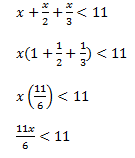x  < 6
Solution set =(–∞, 6).
Solution 10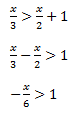x < -6
Solution set =(–∞, –6).
Solution  11: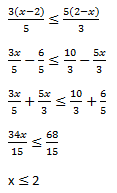Solution set =(–∞, 2].
Solution 12: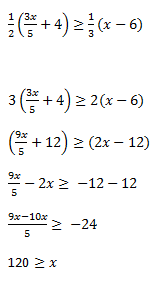Solution set =(–∞, 120].
Solution 13:
2(2x + 3) – 10 < 6 (x – 2)
4x+6-10 <6x-12
-4+12 < 6x-4x
8< 2x
4< x
Solution set =[4, ∞).
Solution 14
37 – (3x + 5) ≥ 9x – 8(x – 3)
37 -3x-5 ≥ 9x – 8x + 24
32 -24 > x+3x
8 > 4x
2 > x
Solution set =(–∞, 2].
Solution 15: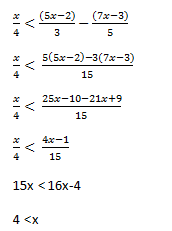Solution set =(4, ∞).
Solution 16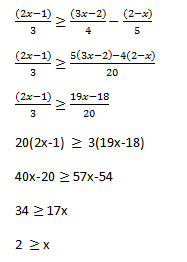Solution set =(–∞, 2].
Solution 17
3x – 2 < 2x +1
⇒ 3x – 2x < 1 + 2
⇒ x < 3
The graphical representation of the solutions of the given inequality is as follows.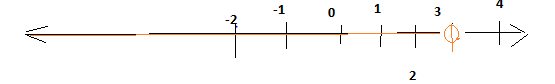Solution  18:
5x – 3 ≥ 3x – 5
2x ≥ -2
x ≥ -1
The graphical representation of the solutions of the given inequality is as follows.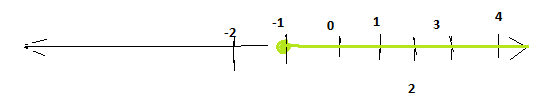Solution 19
3(1 – x) < 2 (x + 4)
3-3x < 2x+8
3-8 < 5x
-1 < x
The graphical representation of the solutions of the given inequality is as follows.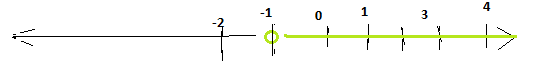Solution 20: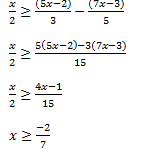Solution 21:
Let x be the marks obtained by Ravi in the third unit test.
Since the student should have an average of at least 60 marks,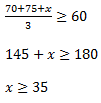Thus, the student must obtain a minimum of 35 marks to have an average of at least 60 marks.
Solution 22:
Let x be the marks obtained by Sunita in the fifth examination.
In order to receive grade ‘A’ in the course, she must obtain an average of 90 marks or
more in five examinations.
Therefore,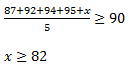Thus, Sunita must obtain greater than or equal to 82 marks in the fifth examination.
Solution 23:
Let y be the smaller of the two consecutive odd positive integers.
Then, the other integer is y + 2.
Since both the integers are smaller than 10,
y + 2 < 10
⇒ y < 10 – 2
⇒ y < 8 … (i)
Also, the sum of the two integers is more than 11.
∴y + (y + 2) > 11
2y + 2 > 11
2y > 11 – 2
2y > 9
From (i) and (ii), we obtain .
Since y is an odd number, y can take the values, 5 and 7.
Thus, the required possible pairs are (5, 7) and (7, 9).
Solution 24:
Let y be the smaller of the two consecutive even positive integers. Then, the other integer
is y + 2.
Since both the integers are larger than 5,
y > 5
Also, the sum of the two integers is less than 23.
y + (y + 2) < 23
2y + 2 < 23
2y < 23 – 2
2y < 21
y < 10.5
But we know  that y > 5
So
5 < y < 10.5.
Since y is an even number, y can take the values, 6, 8, and 10.
Thus, the required possible pairs are (6, 8), (8, 10), and (10, 12).
Solution 25:
Let the length of the shortest side of the triangle be z cm.
Then, length of the longest side = 3z cm
Length of the third side = (3z – 2) cm
Since the perimeter of the triangle is at least 61 cm,
Thus, the minimum length of the shortest side is 9 cm.
Solution 26:
Let the length of the shortest piece be z cm. Then, length of the second piece and the
third piece are (z+ 3) cm and 2z cm respectively.
Since the three lengths are to be cut from a single piece of board of length 91 cm,
z + (z + 3) + 2z cm ≤ 91 cm
4z + 3 ≤ 91
4z ≤ 91 – 3
4z ≤ 88
z  ≤ 22
Also, the third piece is at least 5 cm longer than the second piece.
∴2z ≥ (z + 3) + 5
2z ≥ z + 8
z ≥ 8
From both the results
8 ≤ z ≤ 22
Thus, the possible length of the shortest board is greater than or equal to 8 cm but less
than or equal to 22 cm.